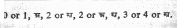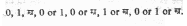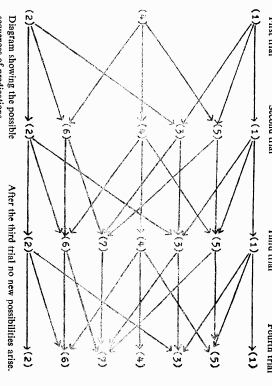## First Steps To Jainism (Part-2)

SANCHETI ASOO LAL
BHANDARI MANAK MAL

By J. B. S. Haldane

The search for truth by the scientific method does not lead to complete certainty. Still less does it lead to complete uncertainty. Hence any logical system which allows of conclusions intermediate between certainty and uncertainty should interest scientists. The earliest such system known to me is the Syadvada system of the Jaina philosopher Bhadrabahu (?433-357 B.C.). Mahalanobis (1954) has commented on it. A central feature of this system is the saptabhanginaya or list of seven types of predication. These are as follows.

(1) syadasti May be it is.

(2) syatnasti May be it is not.

(3) syadastinasti ca. May be it is and is not.

(4) syadavaktavyah. May be it is indeterminate

(5) syadasti ca avaktavyasca. May be it is and is indeterminate.

(6) syatnasti ca avaktavyasca. May be it is not and is indeterminate.

(7) syadasti nasti ca avaktavyasca. May be it is, is not, and is indeterminate

Mahalanobis illustrated this from the throw of a coin, and held that it could serve as a foundation for statistics. However I wish to show that it arises naturally in simpler cases, including simple cases where the affirmative predication asti would be “This is hot”, or “This is a man”.

In any such case an uncertain judgement is usually somewhat quantitative, as in “I think this is a man, though it may be a statue.” I therefore begin with a very abstract field, that of algebra. Here we may be certain of our answer. If x+2=3, then x=1. But if x2-3x+2=0, then x=1 or 2. We cannot say that the probability that x=1 is greater than, less than, or equal to the probability that x=2. Further data may lead to either of these judgements. Five hundred years ago one might perhaps have spoken of indeterminate solutions of equations. Thus if x3 – x2 + x – 1=0, x=1 or + -1. The last two solutions were avakta (incapable of being spoken) until the invention of complex numbers. Today we can find better examples in the field of finite arithmetic.

Consider the finite arithmetic module m. The only admissible values of a variable are the m residues 0, 1, 2, …m -2, m -1, that is to say the possible remainders after division by m. For example module 5, 4+3=7=5+2, so we write 4+3=2. And 4×3 =12=2×5+2, so we write 4×3=2. Let us consider the theory of functions module m. We can define any function f(x) by a table of the values which it assumes for the different admissible values of x. Thus the function 3x (mod.5) can be defined by the table 0, 3, 1, 4, 2. For example if x=4, 3x=2. Of course many other functions are identical with it. For example 3×5=x9+2×5=3x. A function which assumes all the admissible values unequivocally is called biunivocal1 , and it is easy to show that there are m! biunivocal functions. However some functions are univocal, but their inverses are not. In this case some residues do not occur in the table, while others occur more than once. For example the table 3×2+1 (mod. 5) is 1, 4, 3, 3, 4. The number of univocal functions is mm, since each place in the table can be filled in m ways.

If a function is not univocal, but its inverse is univocal, we obtain a table such as that for —1/2, namely 0, 1 or 4, –, –, 1 or 4. Here I introduce the symbol –, for avakta, for an undefined number. There is no number whose square (mod 5) is 2 or 3. — may occur in a table as an alternative to a number. For example the function ex is never integral when x is a residue other than zero. Nor is it integral for most values of x which are avakta, such as –2. But it is integral for such numbers as log 2. Hence the table of ex is 1; –, –, –, –; and 0, 1, 2, 3, 4, or –. The last place in the table corresponds to x=–. Similarly if we consider the function y defined by y3-y2=x2, then we find the tableFor when x=2 or 3, y3-y2-4=0, so y=2 or 2+ 2, the latter two roots being congruent with 1/2 (-1+ -7). These quantities are inexpressible (avakta) module 5. And when x=–, x2=2,3 or –, so y may be 3 or 4, as well as –.

Thus for a full enumeration of functions module m we need a table with m+1 places corresponding to the residues 0, 1, 2 -, m-1, and –. In each place we can set one, or any number, of these symbols, but we must set at least one. So each place can be filled in 2m+1-1 ways, for each of the m+1 symbols can be present or absent, except that all cannot be absent. Thus the total number of functions module m is (2m+1-1)m+1, for example 62, 523, 502, 209 if m=5, as compared with only 120 biunivocal functions, and 3125 univocal.

Now consider the simplest of the finite arithmetic’s, namely arithmetic module 2. There are only two elements, 0 and 1 Electronic calculators are based on this arithmetic. These machines are so designed that each unit, as the result of any instruction, will be active (1) or inactive (0) at any given moment. And it is possible, in principle, to predict whether it will be active or inactive. That is to say ambiguity is avoided, and the machine is designed to operate in terms of univocal functions Nevertheless it is possible to provide such a machine with an instruction to which it cannot given an instruction equivalent to one of the paradoxes of Principia Mathematical, come to no conclusion, but print 101010…. indefinitely. Clearly a machine could be designed to print — in such a case. It is obviously possible to design a machine which would print “0 or 1” in response to the instruction x2-x=0. A machine with the further refinement suggested above would respond “0, 1, or –” to the instruction (x2-x) cos x= 0(mod 2)”. Such a machine could give any of 7 responses, namely :These are the saptabhanghinaya with the omission of the syllable syad.

I now pass to an example where the saptabhanghinaya is actually applied in scientific research, and which I suspect is not far from what was in Bhadrabahu’s mind. In the study of the physiology of the sense organs it is important to determine a threshold. For example a light cannot be seen below a certain intensity, or a solution of a substance which is tasted as bitter when concentrated cannot be distinguished from water when it is diluted. Some experimenters order their subjects to answer “yes” or “no” to the question “Is this illuminated ?” or “Is this bitter ?” If the experimenter is interested in the psychology of perception he will permit the subject also to answer “It is uncertain”, or some equivalent phrase. The objection to this is that some subjects may do so over a wide range of intensities.

Now consider a subject who is shown a series of illuminated patches, some above his threshold of perception, some below it, and others very close to it, in a randomised series. We will suppose that he is in a steady state of sensory adaptation, that he replies in Sanskrit and that he is aware that his answers will sometimes be incorrect. At any given trial he will answer “syadasti”, “syadavaktavyah”, or “syatnasti”. After the second trial of a light of an intensity near the threshold he may have given two of these answers, for example “syatnasti ca avaktavyasca”. After the third he may have given all three, though this is not very probable. The possibilities may be schematised as follows :It is clear that the seven possibilities are exhaustive. My only possible criticism of Bhadrabahu is this. If the subject has given two different answers he may be aware that there is a possibility that he will later give the third. Once he has given all three, no further possibility is open. It might therefore be argued that the seventh of predication should be “asti nasti ca avaktavyasca”.

On the hypothesis that the subject is in a steady physiological and psychological state, the probabilities of each of the three answers to any given stimulus are constant. Let the probabilities of answering syadasti, syadavaktavyah and syatnasti be p, q, and r, where p-q+1=1. If, after n trials, the probabilities of the 7 types of predication are P1,n, P2,n, etc. where P1,n, is the probability of syadasti, etc. then the vector [P1, n, ;P2,n ; P3,n; P4,n; P6,n; P7,n] is transformed into the vector [P1, n+1; P2, n+1; ….. etc.] by multiplication by the matrix.

p 0 0 0 0 0 0

0 r 0 0 0 0 0

r p p+r 0 0 0 0

0 0 0 q 0 0 0

q 0 0 p p+q 0 0

0 q 0 r 0 q+r 0

0 0 q 0 r p 1

Evidently this could be made a little more symmetrical by transposing row and column (3) and (4).

The latent roots of this matrix are :

1, p+q, q+r, p+r, p, q, r.

so P1n = pn

P2n= rn

P3n= (p+r)n-pn-rn

P4n= qn

P5n=(p+q)n-pn-qn

P6n# (q+r)n-qn-rn

P7n=1-(q+r)n-(r+p)n-(q+r)n+pn+qn+rn.

Thus unless one of p,q or r is zero the final predication will be syadasti nasti ca avaktavyasca. In many cases when the stimulus is far from the threshold, p or r will unity. The subject will always, or never, say `this is bitter’, or “this is illuminated”, It is likely that q will ever be unity. So in this case syadavaktavyah will almost always be at best a provisional predication. It is however possible that p or r (say r) should be small, but not zero. If so P5,n will reach a maximum for some value of n and then decline. For example if p=.6, q=.3, r=.1 reaches its maximum value of .5184 when n=4.

I have dealt with a case which arises when the question asked is as simple as possible. Human judgements are generally more complicated. We may attend to the data of several different senses, and of our memories. Thus we arrive at one conclusion from one set of data, and another from another set. We say that wood is hard when compared with clay, soft when compared with iron, indeterminate when compared with similar wood.

The close analysis of vision with a dark adapted eye shows that in this case at least. Mahalanobis was correct in regarding the saptabhanginaya as foreshadowing modern statistical theory. It appears that when dark adaptation is complete, about five quanta of radiation must arrive within a short time in a small area of the retina before light is reported. Whether they will do so with a given intensity of illuminationn can only be stated as a probability. It is probable, though not by any means certain, that more complicated judgements depend on similar probabilities of events within the central nervous system.

Whatever philosophers of other schools may think, a Jaina can hardly object to regarding human predication as a special kind of animal behaviour. In this he agree with followers of Darwin, such as myself. Attempts at a logical classification of animal behaviours frequently lead to a separation of 2n-1 types, where however n may exceed 3. Thus Haldane (1953) classified the possible results of learning in an animal as follows. In any situation an animal will, or will not, give a certain response R, say eating a particular type of food within a minute of its presentation, or lifting its leg within ten seconds after an auditory signal is given.

If we compare the set of possible situations in which an animal may be placed before and after an experience E, they fall into four categories, r r, rR, R r, and RR. A situation r r is one in which the response is not given before or after experience. A situation r R is one which it is given after E, but not before E, and so on. All situation may be r r. For example no-one has taught a dog to write. Some may be r r and some rR. For example a dog which did not previously bring objects from the water to his master can learn to do so on command. In Pavlov’s experiments a dog which previously only salivated (R) when given food, will do so when certain auditory or other stimuli are given. Thus for such a dog all situations fall into the classes r r, RR, and rR. It can easily be seen that the effect of any experience on an animal can be classified according as the situations in which it can be placed fall into one, two, three, or all four of these classes. There are thus 24-1, or 15 qualitatively different results of an experiment in which an attempt is made to alter an animal’s behaviours. In this classification the animal is assumed never to give an indeterminate response. If it can do so, both before and after, there are, as I pointed our, 29-1, or 511 possible results. The same principles may be applied to the comparison of the behaviour of two different animals, or two different races or species.

It is foolish to pretend that ancient philosophers anticipated all modern intellectual developments. And I believe that we, today, can do more honour to their memories by thinking for ourselves, as they did, than by devoting our lives to commentaries on them. But if we do so it is our duty to point out cases where it turns out that our own thought has run parallel to theirs. I was unaware of Bhadrabahu’s existence when I wrote the paper to which I refer. The fact that I reached a conclusion so like his own suggests that we may both have seen the same facet of many-splendoured truth.

No doubt we reached it by very different methods, Bhadrabahu by meditation, I by thinking about the results of concrete experiments on animals. Such methods will often lead to different conclusions. This was the view of Warren Hastings in his introduction to `Wilkins’ translation of the Bhagavad Gita.

“But if we are told that there have been men who were successively, for ages past, in the daily habit of abstracted contemplation, begun in the earliest period of youth, and continued in many to the maturity of age, each adding some portion of knowledge to the store accumulated by his predecessors, it is not assuming too much to conclude, that as the mind ever gathers strength, like the body, with exercise, so in such exercise it may in each have acquired the faculty to which they aspired, and their collective studies have led them to the discovery of new tracks and combinations of sentiment, totally different from the doctrines with which the learned of other nations are acquainted : doctrines, which however speculative and subtle, still, as they possess the advantage of being derived from a source so free from every adventitious mixture, may be equally founded on truth with the most simple of our own”.

If, on the other hand, the contemplation of one’s own mind, and that of the minds of animals, lead to similar results, such results are, perhaps worthy of serious consideration.

References

Mahalanobis, P.C. (1954) : The foundations of statistics Dialectica 8, 95-111.

Haldane, J.B.S. (1954) : A logical analysis of learning, conditioning, and related processes.Behaviour, 6, 256-270.